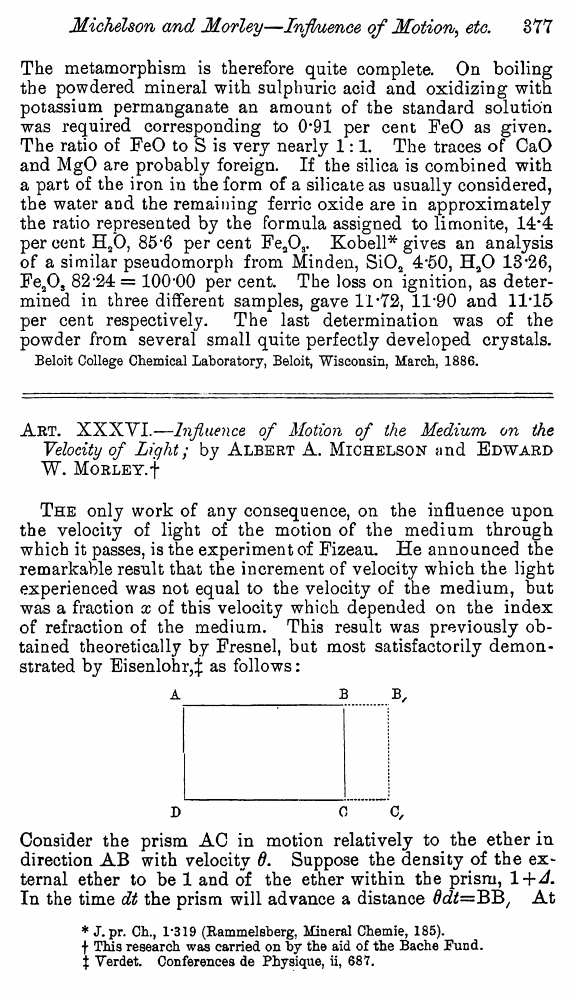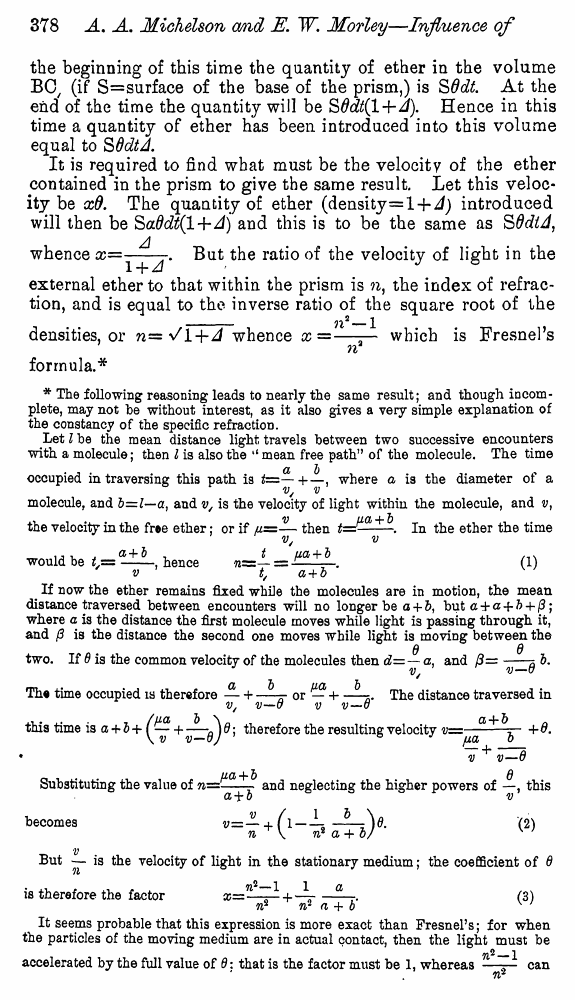﻿ Michelson A.A., Morley E. Influence of Motion of the Medium on the velocity of Light. // American Journal of Science, Ser. 3, 1886, vol. 31

# Michelson A.A., Morley E. Influence of Motion of the Medium on the velocity of Light. // American Journal of Science, Ser. 3, 1886, vol. 31

В начало   Другие форматы   <<<     Страница 377   >>>377 378  379  380  381  382  383  384  385  386 Art. XXXVI.—Influence of Motion of the Medium on the Velocity of Light; by Albert A. Michelson and Edward W. Morley. † The only work of any consequence, on the influence upon the velocity of light of the motion of the medium through which it passes, is the experiment of Fizeau. He announced the remarkable result that the increment of velocity which the light experienced was not equal to the velocity of the medium, but was a fraction x of this velocity which depended on the index of refraction of the medium. This result was previously obtained theoretically by Fresnel, but most satisfactorily demonstrated by Eisenlohr,‡ as follows: A_B B, D 0 C, Consider the prism AC in motion relatively to the ether in direction AB with velocity θ. Suppose the density of the external ether to be 1 and of the ether within the prism, 1+Δ. In the time dt the prism will advance a distance θdt=BB1 At † This research was carried on by the aid of the Bache Fund. ‡ Verdet. Conferences de Physique, ii, 687. the beginning of this time the quantity of ether in the volume B0/ (if S=surface of the base of the prism,) is SOdt At the end of the time the quantity will be SOdtil+A). Hence in this time a quantity of ether has been introduced ioto this volume equal to S Odt A. It is required to find what must be the velocity of the ether contained in the prism to give the same result. Let this velocity be xO. The quantity of ether (density=l-J- A) introduced will then be SaOdt(l+A) and this is to be the same as SOdiA, whence X==Y^J' ^ut rat*° ve'oc^y in the external ether to that within the prism is n, the index of refraction, and is equal to the inverse ratio of the square root of the __ji2_1 densities, or n='/l-hA whence x =—— which is Fresnel’s n formula.* * The following reasoning leads to nearly the same result; and though incomplete, may not he without interest, as it also gives a very simple explanation of the constancy of the specific refraction. Let I he the mean distance light travels between two successive encounters with a molecule; then I is also the ‘‘ mean free path” of the molecule. The time occupied in traversing this path is fc=— +—, where a is the diameter of a molecule, and b=l—a, and v/ is the velocity of light within the molecule, and v, the velocity in the free ether: or if ll=— then #=jtza + -. ln the ether the time v/ v would be /,= , hence 71= — = + ^ . (1) ' v t, a + b If now the ether remains fixed while the molecules are in motion, the mean distance traversed between encounters will no longer be a + &, but a + a + b 4- (3; where a is the distance the first molecule moves while light is passing through it, and /? is the distance the second one moves while light is moving between the q d two. If 6 is the common velocity of the molecules then d= — a, and 3=-3 b. The time occupied is therefore — + —r or — H--s<. The distance traversed in v/ v—u v v—o , . . /UOL b \ CL + b 0 this time is a + b + f J Q; therefore the resulting velocity j • v v—B ua + b 6 Substituting the value of n=-r and neglecting the higher powers of —, this a + o ^ becomes v~— + (1—(2) n^\ n* a+ b/ w v But is the velocity of light in the stationary medium; the coefficient of 6 is therefore the factor --(3) n* w a + b w It seems probable that this expression is more exact than Fresnel’s; for when the particles of the moving medium are in actual contact, then the light must be ^2_1 accelerated by the full value of 0: that is the factor must be 1, whereas —— can